08.02.2020 17:55 14.02.2020 12:03
##### Principle and processing:

The example used a C-IF-6400R module equipped with a Grid-EYE sensor, which is 64 precision thermal sensors arranged in an 8x8 matrix. Provides temperature map data at a viewing angle of 60 degrees, horizontally and vertically. Allows measurement 1x or 10x per second. The configuration is performed in the Mosaic development environment, more precisely in the I / O Configurator. The module transmits information from the sensor using 3 parameters - INDEX, REF and TEMP. INDEX defines the line (1 to 8), REF reference temperature and TEMP (8x temperature in tenths of a degree) difference of temperatures of individual sensors from the reference temperature REF.

We will complete the final temperature segment using the formula: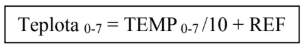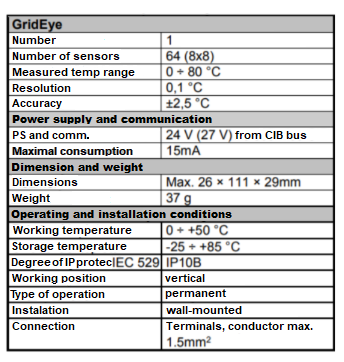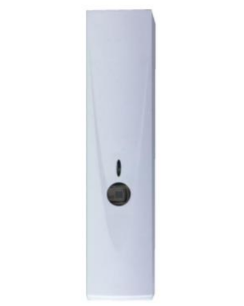The C-IF-6400R sensor was connected to the Foxtrot PLC via the CIB interface, and only a 24V MW DR-60-24 power supply was connected for powering the PLC (the sensor is powered directly from the CIB).

According to the catalog sheet, the above formula and other information about the sensor, an algorithm was first built to calculate the temperature measured by each segment. This forms the largest part of the text in the program, as it was necessary to break down the program for each line of segments.

The first part simply compares the temperature of the segment with the limit temperature temp_trig, if this temperature is exceeded, the segment turns red, if not, it turns blue. The limit temperature can be set in the web interface. The segment map is in the web interface to the right of the controls. This map is also used to detect people.

Possible temperatures (with respect to possible outputs and measurements) were divided into several intervals, which were assigned values 0 - 7, according to which the color of the segments (multi-state image) was set in the web interface, which forms a smaller map on the right. White means the warmest, purple means the coldest. The colors are for guidance only. This map is only 6x6 (missing margins), as the possible number of variables in the web interface would be exceeded.

A person is detected in the case of 2 active segments one above the other, ie when the temperature of these two segments exceeds the limit. This selection was chosen according to the results of several measurements.

The next part of the program verifies whether the person is on the right or left side of the scanned field. Accordingly, the corresponding indicator in the web interface lights up.

In the resulting program, the coordinates of the edges of the area in which the human presence will be detected are entered at the beginning. The coordinates are given by the sequence number of the corner segment, the order of the ABCD vertices (corn_a, corn_b,…) is given by the standard marking of the vertices of the rectangles in the geometry, ie counterclockwise, starting with the lower left corner.

The program first reads the temperatures, compares them with the boundary and creates a temperature map. It then examines this temperature map with the FOR cycle and looks for 2 active segments on top of each other (originally there were 3, but it was not functional for a greater distance). If it finds at least one pair, the LED on the sensor and on the web will light up. the right / left indicator also lights up on the interface. It is then determined whether or not the three segments are in the reserved zone. If so, the value of in_zone is set to 1.

The variables led, right, left, in_zone are set back to 0 a few seconds after setting 1, unless a pair of active segments is found.

If a person is detected on the map but remains outside the reserved field for approximately 10 seconds, the orange WARN indicator lights up, which must then be reset manually. This function is switched by the switch to the left of WARN.

Web interface:

Limit temperature - the temperature at which one segment is activated, the temperature is optimally determined after testing in the given environment

Zone borders - the four "coordinates" defining the rectangle in which the human presence will be required must be chosen so that the sides of the rectangle are vertical and horizontal, the coordinates are given by the serial number of the corner segment

Confirm button - sends the newly entered zone boundaries

LED - indicates the presence of a person, corresponds to the status of the diode located on the sensor

LFT/RGHT – indication of human presence in the left / right half of the map

ZONE – indication of human presence in the reserved zone

OFF/ON – switch for the function of warning the presence of a person outside the zone

WARN – it is activated when there is a longer human presence outside the reserved zone

RESET – resets WARN to zero

The first image shows us the look of the web. interface in the absence of a human in front of the sensor.

The second image shows us the look of the web. interface when a person is standing close to the sensor, at least part of the reserved zone and to the right.

In the third image, the detection of a person outside the zone is turned on and the WARN detection is lit.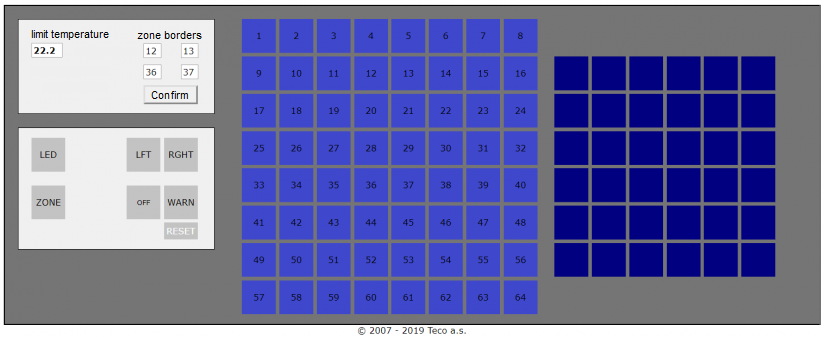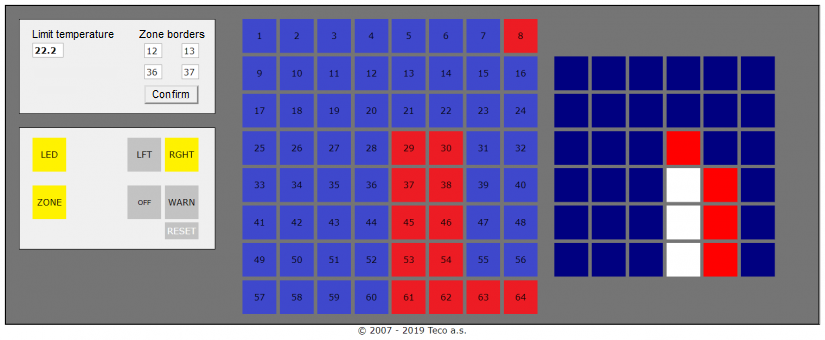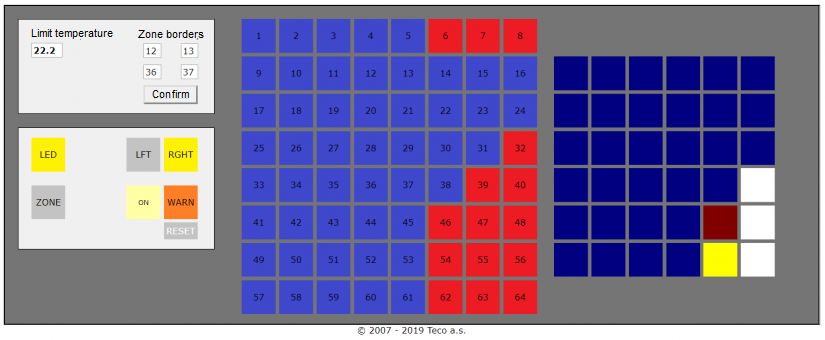Code:

``````PROGRAM prgMain

VAR
i            : INT;
j            : INT;
left, right  : BOOL := 0;
led_delay    : INT := 0;
temp         : ARRAY [1..64] of REAL;
help         : ARRAY [1..8]  of REAL;
temp_1Wx     : REAL;
temp_avg     : REAL;
presence     : ARRAY [1..64] of BOOL;
present      : BOOL := 0;
color_temp   : ARRAY [1..64] of INT;
temp_trig    : REAL := 22.2;
temp_lvl     : ARRAY [0..7] of REAL := [22.2,22.5,22.7,23.0,23.2,23.5,23.7,24.0];
warn_on      : BOOL := 0;

// rectangular zone position - ABCD rectangle from the lower left corner
corn_a       : INT := 36;
corn_b       : INT := 37;
corn_c       : INT := 13;
corn_d       : INT := 12;

//dimesions
a,b          : INT;
in_zone      : BOOL:= 0;
warn         : BOOL:= 0;
warn_delay   : INT := 0;

END_VAR

//rectangle calculation
b := (corn_a - corn_d)/8 + 1;
a := (corn_b - corn_a) + 1;

//saving the temperature to the map
FOR i := 0 to 7 do
help[i+1] := SINT_TO_REAL(col[i]);
END_FOR;

IF line = 0 then
FOR i := 1 to 8 do
temp[i] := help[i] / 10.0 + ref;
IF temp[i] <= temp_lvl then
color_temp[i] := 0;
ELSIF temp[i] >= temp_lvl then
color_temp[i] := 7;
END_IF;
FOR j := 1 to 6 do
IF temp[i] = temp_lvl[j] then
color_temp[i] := j;
END_IF;
END_FOR;
END_FOR;

ELSIF line = 1 then
FOR i := 1 to 8 do
temp[i+8] := help[i] / 10.0 + ref;
IF temp[i+8] <= temp_lvl then
color_temp[i+8] := 0;
ELSIF temp[i+8] >= temp_lvl then
color_temp[i+8] := 7;
END_IF;
FOR j := 1 to 6 do
IF temp[i+8] = temp_lvl[j] then
color_temp[i+8] := j;
END_IF;
END_FOR;
END_FOR;

ELSIF line = 2 then
FOR i := 1 to 8 do
temp[i+16] := help[i] / 10.0 + ref;
IF temp[i+16] <= temp_lvl then
color_temp[i+16] := 0;
ELSIF temp[i+16] >= temp_lvl then
color_temp[i+16] := 7;
END_IF;
FOR j := 1 to 6 do
IF temp[i+16] = temp_lvl[j] then
color_temp[i+16] := j;
END_IF;
END_FOR;
END_FOR;

ELSIF line = 3 then
FOR i := 1 to 8 do
temp[i+24] := help[i] / 10.0 + ref;
IF temp[i+24] <= temp_lvl then
color_temp[i+24] := 0;
ELSIF temp[i+24] >= temp_lvl then
color_temp[i+24] := 7;
END_IF;
FOR j := 1 to 6 do
IF temp[i+24] = temp_lvl[j] then
color_temp[i+24] := j;
END_IF;
END_FOR;
END_FOR;

ELSIF line = 4 then
FOR i := 1 to 8 do
temp[i+32] := help[i] / 10.0 + ref;
IF temp[i+32] <= temp_lvl then
color_temp[i+32] := 0;
ELSIF temp[i+32] >= temp_lvl then
color_temp[i+32] := 7;
END_IF;
FOR j := 1 to 6 do
IF temp[i+32] = temp_lvl[j] then
color_temp[i+32] := j;
END_IF;
END_FOR;

END_FOR;

ELSIF line = 5 then

FOR i := 1 to 8 do

temp[i+40] := help[i] / 10.0 + ref;

IF temp[i+40] <= temp_lvl then

color_temp[i+40] := 0;

ELSIF temp[i+40] >= temp_lvl then

color_temp[i+40] := 7;

END_IF;

FOR j := 1 to 6 do

IF temp[i+40] = temp_lvl[j] then

color_temp[i+40] := j;

END_IF;

END_FOR;

END_FOR;

ELSIF line = 6 then

FOR i := 1 to 8 do

temp[i+48] := help[i] / 10.0 + ref;

IF temp[i+48] <= temp_lvl then

color_temp[i+48] := 0;

ELSIF temp[i+48] >= temp_lvl then

color_temp[i+48] := 7;

END_IF;

FOR j := 1 to 6 do

IF temp[i+48] = temp_lvl[j] then

color_temp[i+48] := j;

END_IF;

END_FOR;

END_FOR;

ELSIF line = 7 then

FOR i := 1 to 8 do

temp[i+56] := help[i] / 10.0 + ref;

IF temp[i+56] <= temp_lvl then

color_temp[i+56] := 0;

ELSIF temp[i+56] >= temp_lvl then

color_temp[i+56] := 7;

END_IF;

FOR j := 1 to 6 do

IF temp[i+56] = temp_lvl[j] then

color_temp[i+56] := j;

END_IF;

END_FOR;

END_FOR;

END_IF;

//search and marking of segments with higher than the limit temperature

FOR i := 1 to 64 do

IF temp[i] >= temp_trig then

presence[i] := 1;

ELSIF temp[i] < temp_trig then

presence[i] := 0;

END_IF;

END_FOR;

FOR i := 1 to 48 do

IF presence[i] AND presence[i+8] then //AND presence[i+16] then  //only active 3 segments on top of each other feel like human presence - elimination of interference / anomalies / airflow

led := 1;

present := 1;

IF (i >= 1 AND i <= 4) OR (i >= 9 AND i <= 12) OR (i >= 17 AND i <= 20) OR  //divided into right and left side

(i >= 25 AND i <= 28) OR (i >= 33 AND i <= 36) OR (i >= 41 AND i <=44) OR (i >= 49 AND i <=52) then

left := 1;

ELSE right := 1;

END_IF;

//detection of a person in a reserved field

FOR j := 0 to (b-2) do

IF (i >= corn_d + 8*j) AND (i <= corn_c + 8*j) then

in_zone := 1;

EXIT;

END_IF;

END_FOR;

END_IF;

END_FOR;

IF led_delay > 300 then

led := 0;

led_delay := 0;

left := 0;

right := 0;

in_zone := 0;

END_IF;

IF led then

led_delay := led_delay + 1;

END_IF;

IF present AND (not in_zone) AND warn_on then

warn_delay := warn_delay + 1;

IF warn_delay >= 1000 then //about 10 seconds

warn := 1;               //the warn will light up

warn_delay := 0;

END_IF;

ELSE

warn_delay := 0;

END_IF;

present := 0;

END_PROGRAM``````